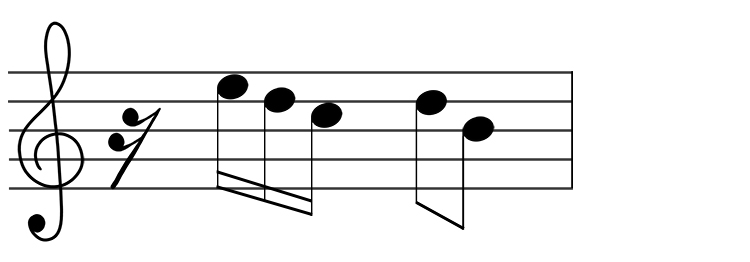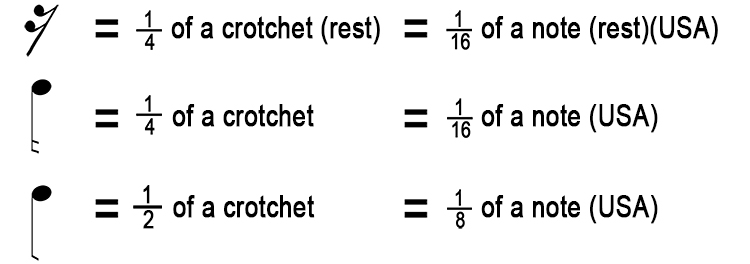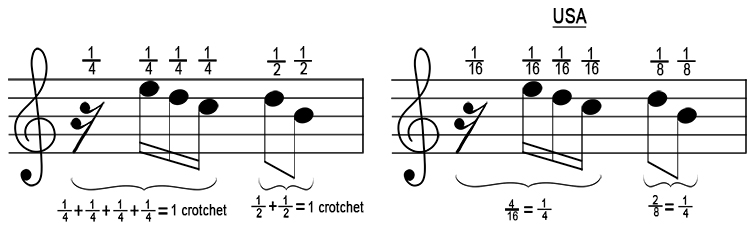# Time Signatures Exam theory questions - example 1

What is the correct time signature?In order to do this you need to break down the value of each note:Then write these values above each note on the bar:There are 2 crotchets in this bar, so the time signature would be:

2/4 = 2  Ncirctimes crotchets = 2  Ncirctimes1/4 notes (USA)

The time signature is 2/4